• ## 使用pandas读取excel

万次阅读 多人点赞 2018-10-30 15:53:01
Excel是微软的经典之作，在日常工作中的数据整理、分析和可视化方面，有其独到的优势，尤其在你熟练应用了函数和数据透视等高级功能之后，Excel可以大幅度提高你的工作效率。但如果数据量超大，Excel的劣势也就随之...
本文为作者原创，未经允许不得擅自转载。

Excel是微软的经典之作，在日常工作中的数据整理、分析和可视化方面，有其独到的优势，尤其在你熟练应用了函数和数据透视等高级功能之后，Excel可以大幅度提高你的工作效率。但如果数据量超大，Excel的劣势也就随之而来，甚至因为内存溢出无法打开文件，后续的分析更是难上加难。那么，有什么更好的解决办法吗？工欲善其事，必先利其器，在这里我们介绍使用Python的pandas数据分析包来解决此问题。

pd.read_excel(io, sheet_name=0, header=0, names=None, index_col=None,
usecols=None, squeeze=False,dtype=None, engine=None,
converters=None, true_values=None, false_values=None,
skiprows=None, nrows=None, na_values=None, parse_dates=False,
date_parser=None, thousands=None, comment=None, skipfooter=0,
convert_float=True, **kwds)


目录

1、io，Excel的存储路径

2、sheet_name，要读取的工作表名称

4、names， 自定义最终的列名

5、index_col， 用作索引的列

6、usecols，需要读取哪些列

7、squeeze，当数据仅包含一列

8、converters ，强制规定列数据类型

9、skiprows，跳过特定行

10、nrows ，需要读取的行数

11、skipfooter ， 跳过末尾n行

【文中使用英超、西甲的排名积分榜及射手榜作为原始数据~~~】

1、io，Excel的存储路径

建议使用英文路径以及英文命名方式。
import pandas as pd
io = r'C:\Users\Administrator\Desktop\data.xlsx'

2、sheet_name，要读取的工作表名称

可以是整型数字、列表名或SheetN，也可以是上述三种组成的列表。

整型数字：目标sheet所在的位置，以0为起始，比如sheet_name = 1代表第2个工作表。

data = pd.read_excel(io, sheet_name = 1)
data.head()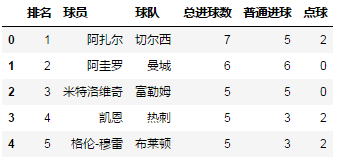列表名：目标sheet的名称，中英文皆可。
data = pd.read_excel(io, sheet_name = '英超射手榜')
data.head()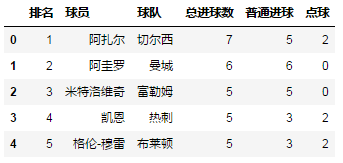SheetN：代表第N个sheet，S要大写，注意与整型数字的区别。
data = pd.read_excel(io, sheet_name = 'Sheet5')
data.head()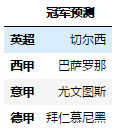组合列表： sheet_name = [0, '英超射手榜', 'Sheet4']，代表读取三个工作表，分别为第1个工作表、名为“英超射手榜”的工作表和第4个工作表。显然，Sheet4未经重命名。
sheet_name 默认为0，取Excel第一个工作表。如果读取多个工作表，则显示表格的字典。对于初学者而言，建议每次读取一个工作表，然后进行二次整合。
data = pd.read_excel(io, sheet_name = ['英超积分榜', '西甲积分榜'], nrows = 5)
# sheet_name = ['英超积分榜', '西甲积分榜'] ，返回两个工作表组成的字典
data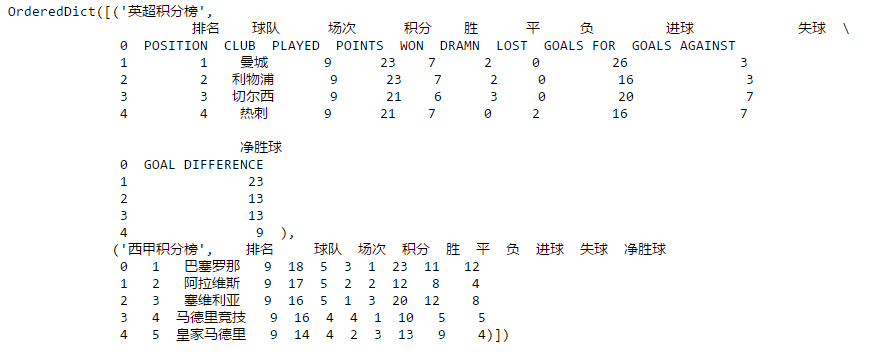默认为0 ，如果设置为[0,1]，则表示将前两行作为多重索引。
data = pd.read_excel(io, sheet_name = '英超积分榜', header = [0,1])
# 前两行作为列名。
data.head()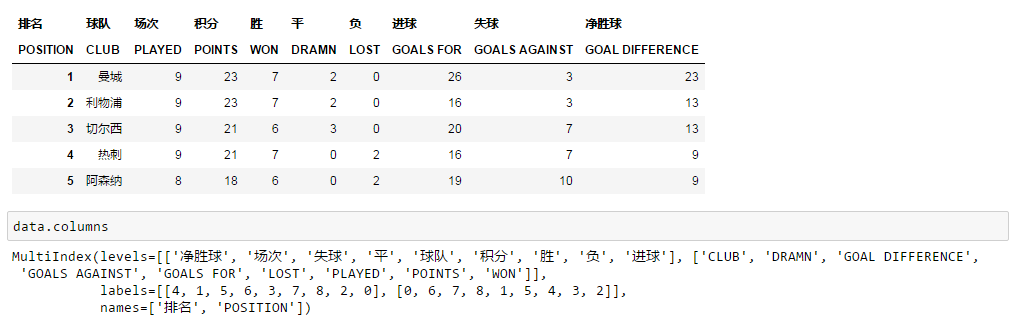4、names， 自定义最终的列名

一般适用于Excel缺少列名，或者需要重新定义列名的情况。
注意：names的长度必须和Excel列长度一致，否则会报错。
data = pd.read_excel(io, sheet_name = '英超射手榜',
names = ['rank','player','club','goal','common_goal','penalty'])
data.head()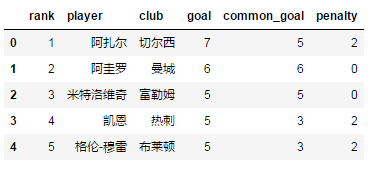5、index_col， 用作索引的列

可以是工作表列名称，如index_col = '排名'；
可以是整型或整型列表，如index_col = 0 或 [0, 1]，如果选择多个列，则返回多重索引。
data = pd.read_excel(io, sheet_name = '英超射手榜', index_col = '排名')
data.head()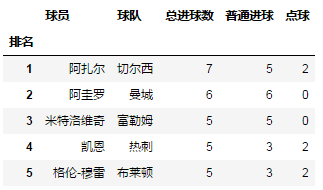data = pd.read_excel(io, sheet_name = '英超射手榜', index_col = [0, 1])
data.head()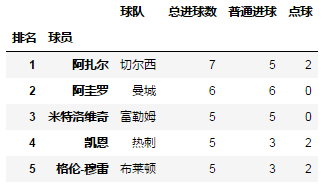6、usecols，需要读取哪些列

可以使用整型，从0开始，如[0,2,3]；
可以使用Excel传统的列名“A”、“B”等字母，如“A：C, E” ="A, B, C, E"，注意两边都包括。
usecols 可避免读取全量数据，而是以分析需求为导向选择特定数据，可以大幅提高效率。
data = pd.read_excel(io, sheet_name = '西甲射手榜', usecols = [0, 1, 3])
data.head()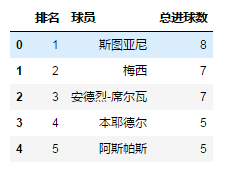data = pd.read_excel(io, sheet_name = '西甲射手榜', usecols = 'A:C, E')
# 啊？什么！！为啥不见C罗？？
# 大佬，C罗转会去尤文图斯啦~~~~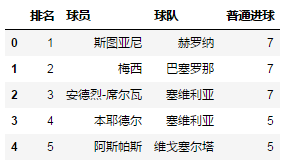7、squeeze，当数据仅包含一列

squeeze为True时，返回Series，反之返回DataFrame。
data = pd.read_excel(io, sheet_name = 'squeeze', squeeze  = True)
data.head()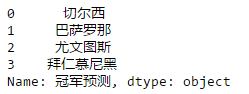data = pd.read_excel(io, sheet_name = 'squeeze', squeeze  = False)
data.head()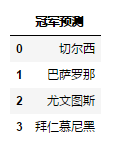8、converters ，强制规定列数据类型

converters = {'排名': str, '场次': int}， 将“排名”列数据类型强制规定为字符串（pandas默认将文本类的数据读取为整型），“场次”列强制规定为整型；

主要用途：保留以文本形式存储的数字。

data = pd.read_excel(io, sheet_name = 'converters')
data['排名'].dtype

data = pd.read_excel(io, sheet_name = 'converters', converters = {'排名': str, '场次': float})
data['排名'].dtype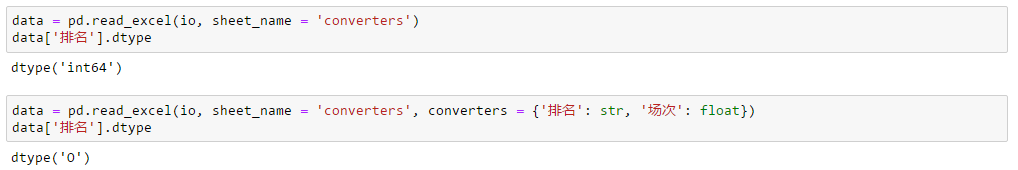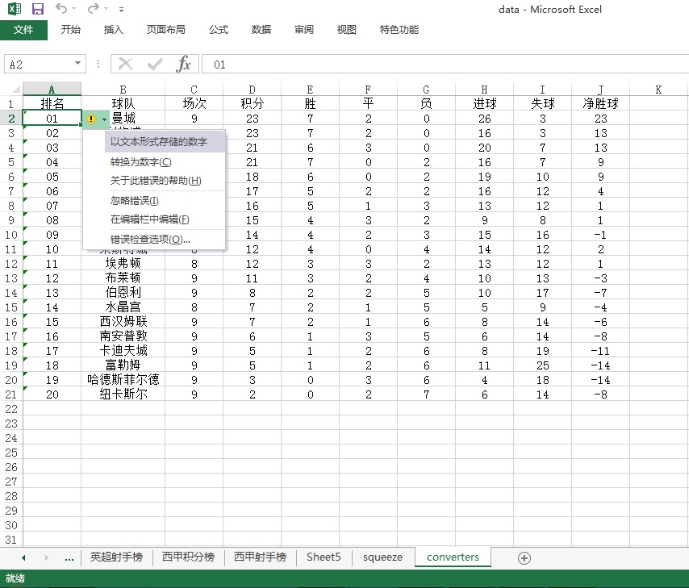9、skiprows，跳过特定行

skiprows= n， 跳过前n行； skiprows = [a, b, c]，跳过第a+1,b+1,c+1行（索引从0开始）；
使用skiprows 后，有可能首行（即列名）也会被跳过。
data = pd.read_excel(io, sheet_name = '英超射手榜', skiprows = [1,2,3])
# 跳过第2,3,4行数据（索引从0开始，包括列名）
data.head()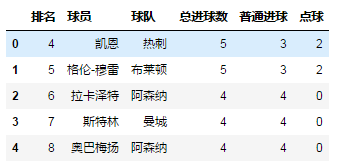data = pd.read_excel(io, sheet_name = '英超射手榜', skiprows = 3)
data.head()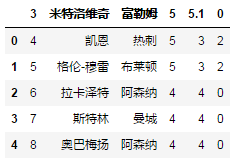10、nrows ，需要读取的行数

如果只想了解Excel的列名及概况，不必读取全量数据，nrows会十分有用。
data = pd.read_excel(io, sheet_name = '英超射手榜', nrows = 10)
data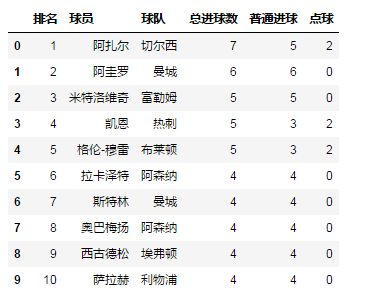11、skipfooter ， 跳过末尾n行

data = pd.read_excel(r'C:\Users\Administrator\Desktop\data.xlsx' ,
sheet_name = '英超射手榜', skipfooter = 43)
#  skipfooter = 43， 跳过末尾43行（索引从0开始）
data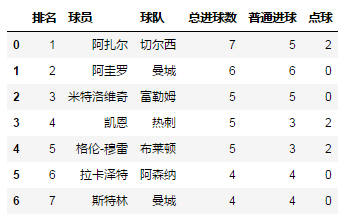欢迎搜索今日头条“海阔天空爱阅读”，欣赏更多文章~~

展开全文Python
• 主要介绍了解决python pandas读取excel多个不同sheet表格存在的问题，具有很好的参考价值，希望对大家有所帮助。一起跟随小编过来看看吧
• 一、背景 用python（2.7） pandas处理excel多个sheet，各sheet之间有关联字段，起到的效果相当于多张RDB的表，做联表查询。 二、da
一、背景

用python（2.7） pandas处理excel多个sheet，各sheet之间有关联字段，起到的效果相当于多张RDB的表，做联表查询。

二、python代码

import pandas as pd
import xlsxwriter

def print_hi(name):
# Use a breakpoint in the code line below to debug your script.
dfCity = \
sheet_name='city')
dfArea = \
sheet_name='area')
dfHospital = \
sheet_name='hospital')
dfDepartment = \
sheet_name='department')
dfDoctor = \
sheet_name='doctor')

df1 = pd.merge(dfCity, dfArea, left_on='id_city', right_on='cityId', how='inner')
df2 = pd.merge(df1, dfHospital, left_on='id_area', right_on='areaId', how='inner')
df3 = pd.merge(df2, dfDepartment, left_on='id_hospital', right_on='hospitalId', how='inner')
df4 = pd.merge(df3, dfDoctor, left_on='id_department', right_on='departmentId', how='inner')

with pd.ExcelWriter('/Users/xxxx/Documents/excels/doctor_data_merge.xlsx',
engine='xlsxwriter',
options={'strings_to_urls': False}) as writer:
df4.to_excel(writer, index=False)

if __name__ == '__main__':
print_hi('PyCharm')


展开全文python
• pandas 的DataFrame 数据结构与excel中的数据形式极为相似，因此对于excel数据的读取和输出使用pandas再适合不过了，因此就涉及到了pandas对于excel文件的读取、写入一个sheet、写入多个sheet、追加sheet等操作。
本文全部操作只需要引入pandas包即可
import pandas as pd

一、生成excel文件
首先构造pandas的DataFrame数据：
data = pd.DataFrame(
{"col1":[1, 2, 3],
"col2":[4, 5, 6],
"col3":[7, 8, 9]
}
)

数据如下：
In :data
Out:
col1  col2  col3
0     1     4     7
1     2     5     8
2     3     6     9

这跟excel的行列展示极为相似。
然后就是使用pandas的to_excel方法生成excel文件并将该内容写入该excel文件：
data.to_excel("excel 样例.xlsx", index=False)

因为index的选项为False，所以没有将索引写入，结果如下：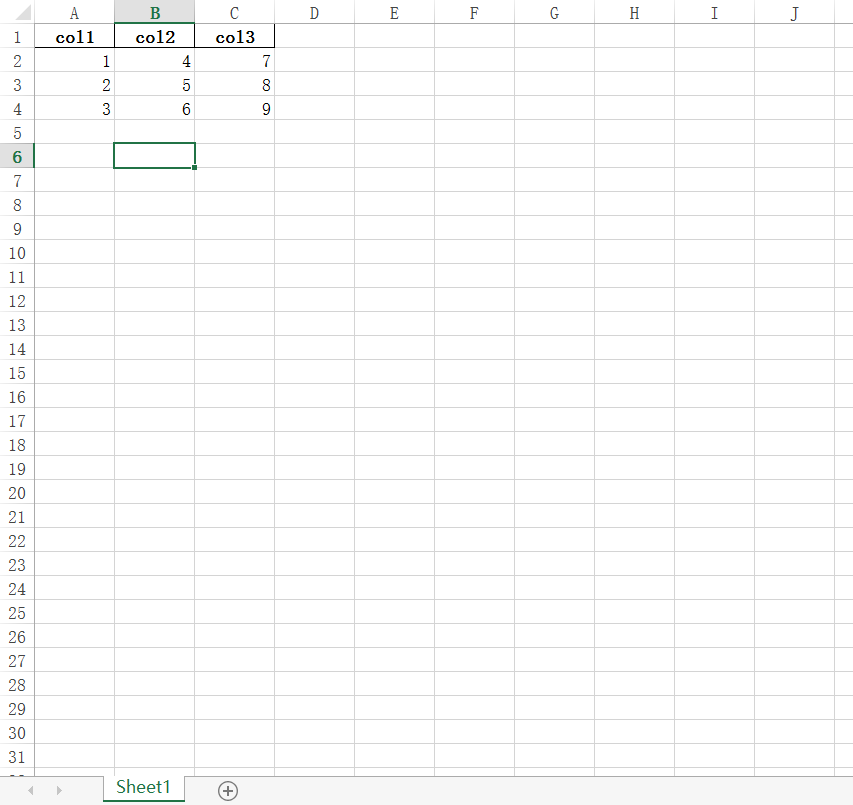同时还可以指定sheet名称、是否展示列名等其他操作，具体可见官网参数说明
二、读取excel文件
data = pd.read_excel("excel 样例.xlsx")

结果与上面创建的结果一样
In :data
Out:
col1  col2  col3
0     1     4     7
1     2     5     8
2     3     6     9

这是最简单的读取excel，同样可以参考官网的参数说明来进行参数设置，指定sheet_name，表头等其他操作。
excel数据里经常会有数据说明、合并项等不需要读取或影响读取数据的单元格，比如下面的样例数据：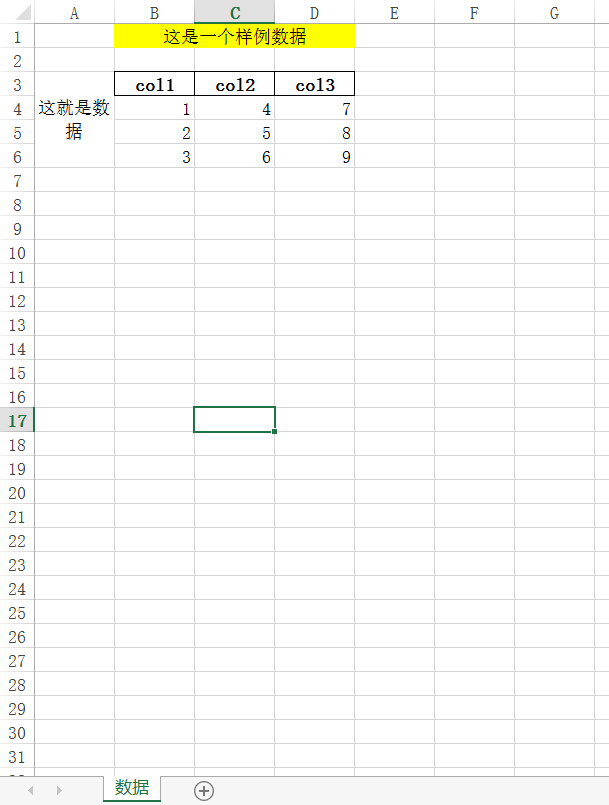那么就要进行表头的设置、初始行的设置、sheet的选择：
data1 = pd.read_excel("excel 样例 1.xlsx", sheet_name="数据", header=2, usecols="B:E")

In :data
Out:
col1  col2  col3
0     1     4     7
1     2     5     8
2     3     6     9

三、一次性插入多个sheet数据
将DataFrame数据写进excel文件中使用的还是文章开头的to_excel方法，但是需要添加引擎writer，如下所示：
data = pd.DataFrame(
{"col1":[1, 2, 3],
"col2":[4, 5, 6],
"col3":[7, 8, 9]
}
)
writer = pd.ExcelWriter("excel 样例.xlsx")
data.to_excel(writer, sheet_name="这是第一个sheet")
data.to_excel(writer, sheet_name="这是第二个sheet")
data.to_excel(writer, sheet_name="这是第三个sheet")
writer.save()
writer.close()

使用pd.ExcelWriter生成writer，然后就可将数据写入该excel文件了，但是写完之后必须要writer.save()和writer.close()，否则数据仍然只在数据流中，并没保存到excel文件中，或者使用with as 魔术方法，这样就会在数据写入完后自动保存并关闭句柄：
with pd.ExcelWriter("excel 样例.xlsx") as writer:
data.to_excel(writer, sheet_name="这是第一个sheet")
data.to_excel(writer, sheet_name="这是第二个sheet")
data.to_excel(writer, sheet_name="这是第三个sheet")

写入的结果如下：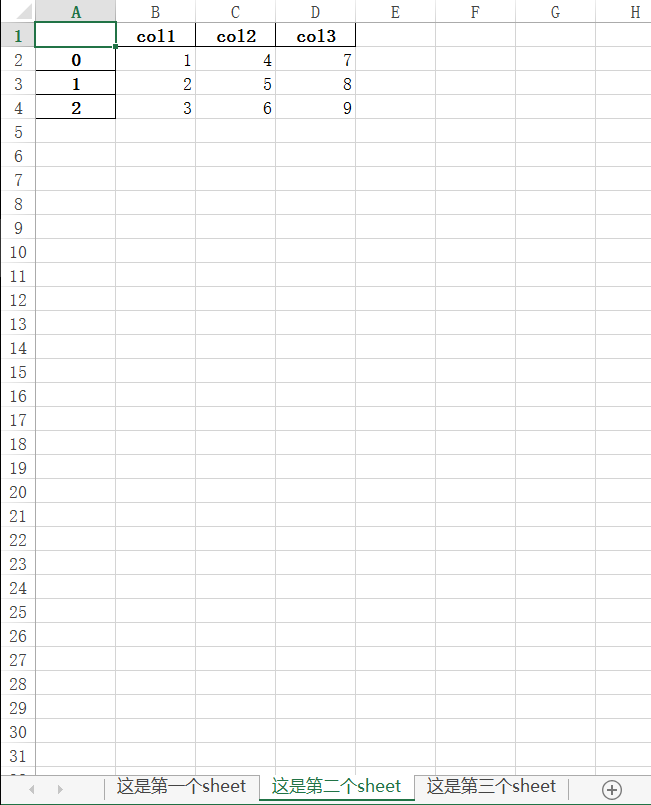注意此操作会将原文件内容覆盖掉，如想追加数据请看下章节

四、追加sheet内容
按照官网的示例使用writer = pd.ExcelWriter("excel 样例.xlsx", mode='a')就能插入sheet，而不是覆盖原文件，然而我进行该操作之后就报错了：
In :writer = pd.ExcelWriter("excel 样例.xlsx", mode='a')
Traceback (most recent call last):

File "<ipython-input-75-8f1e772ce767>", line 1, in <module>
writer = pd.ExcelWriter("excel 样例.xlsx", mode='a')

File "D:\anaconda\lib\site-packages\pandas\io\excel\_xlsxwriter.py", line 177, in __init__
raise ValueError("Append mode is not supported with xlsxwriter!")

ValueError: Append mode is not supported with xlsxwriter!

原因是现在常用的写入excel模块是openpyxl和xlsxwriter，pd.ExcelWriter方法默认是xlsxwriter，但是xlsxwriter不支持append操作，具体解释可以参考这篇博文。因此我们只需要更改模块就行：
data = pd.DataFrame(
{"col1":[1, 2, 3],
"col2":[4, 5, 6],
"col3":[7, 8, 9]
}
)
with pd.ExcelWriter("excel 样例.xlsx", mode='a', engine='openpyxl') as writer:
data.to_excel(writer, sheet_name="这是追加的第1个sheet")
data.to_excel(writer, sheet_name="这是追加的第2个sheet")


结果如下：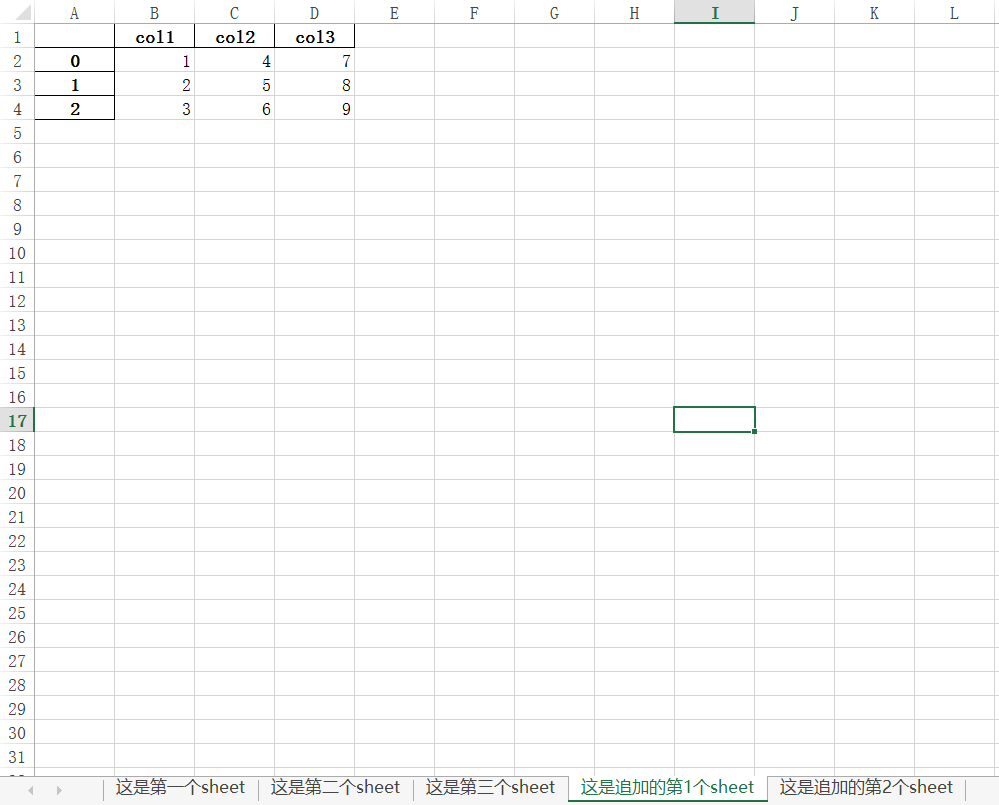最后，还有个原sheet追加内容我没有写入，不写的原因是因为这样的需求太少了，如果实在是有这个需求我也可以通过读取该sheet内容然后使用pd.concat合并数据最后再写入。


展开全文python 插入数据
• 指定某一列作为拆分依据,pandas如何把一个excel的一个sheet拆分为多个sheet呢？具体思路如下：1、读取excel文件获取DataFrame对象df。2、获取指定列的Series对象，去重该指定列得到分类。3、循环分类对df做布尔判断...
指定某一列作为拆分依据,pandas如何把一个excel的一个sheet拆分为多个sheet呢？具体思路如下：1、读取excel文件获取DataFrame对象df。2、获取指定列的Series对象，去重该指定列得到分类。3、循环分类对df做布尔判断写入新的excel。具体实现代码如下：# -*- coding: utf-8 -*-import pandas as pd# 读取exceldf = pd.read_excel('kwd.xlsx')# 去重指定列的类别citys = list(df['城市'].drop_duplicates())with pd.ExcelWriter('kwd_new.xlsx') as excel_writer:# 循环每一类写入for city in citys:bool_df = df['城市'] == citymy_df = df[bool_df]my_df.to_excel(excel_writer,sheet_name=city,index=False)
展开全文• 摘要：不同方法读取excel中的多个不同sheet表格性能比较# 方法1def read_excel(path):df=pd.read_excel(path,None)print(df.keys())# for k,v in df.items():# print(k)# print(v)# print(type(v))return df# 方法2...
• 一、读取多个表单 import pandas as pd excel_reader=pd.ExcelFile('文件.xlsx') # 指定文件 sheet_names = excel_reader.sheet_names # 读取文件的所有表单名，得到列表 df_data = excel_reader.parse(sheet_...
• 摘要：不同方法读取excel中的多个不同sheet表格性能比较# 方法1def read_excel(path):df=pd.read_excel(path,None)print(df.keys())# for k,v in df.items():# print(k)# print(v)# print(type(v))return df# 方法2...
• 摘要：不同方法读取excel中的多个不同sheet表格性能比较 # 方法1 def read_excel(path): df=pd.read_excel(path,None) print(df.keys()) # for k,v in df.items(): # print(k) # print(v) # print(t...
• 摘要：不同方法读取excel中的多个不同sheet表格性能比较# 方法1def read_excel(path):df=pd.read_excel(path,None)print(df.keys())# for k,v in df.items():# print(k)# print(v)# print(type(v))return df# 方法2...
• 读取Excel中不同sheet数据 设置sheet_name=None，会得到一字典变量，字典的key就是sheet名，value就是对应sheet里的数据 import pandas as pd df = pd.read_excel("test.xlsx",sheet_name=None) 不同sheet写入到...python
• pandas读取excel固定的列数 以下为读取excel的第一和第三列 import pandas as pd io=r '路径' data=pd.read_excel(io,usecols=[0,2]) # 指定读取第1列和第3列 data.head() rnows——读取需要的行数 data = pd.read_...python
• pandas读取excel使用 read_excel() 函数 import pandas as pd df = pd.read_excel('goods_base.xlsx') sheet_name 参数, 指定读取 sheet 子表 df = pd.read_excel('sheet_name.xlsx', sheet_name=2) df = pd....
• 一般我们用pandas读取excel时默认都是读入第一个表中的内容，那么如果一个excel有多个sheet(表)，如果按需读取或者全部读取呢？ 数据格式： 读取 默认读取第一张表 data = pd.read_excel('d://测试数据.xlsx...python
• ## pandas读取excel文件

千次阅读 2021-02-23 17:12:40python
• 今天一个不太懂编程的朋友让我帮他处理一些excel的表格，要求大概是：有20多个excel表，每个表里有42个sheet表格（sheet数量和名字相同）。最终需要根据对应的sheet名称将所有表格拼接成一个。 为了方式朋友数据...DataFrame openpyxl
• 原文：【数据处理】pandas DataFrame 对多个sheet表格的excel 读取、处理、保存python...